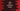# Java program to print the harmonic series## Java program to print harmonic series:

In this post, we will learn how to print the harmonic series in Java. It is the infinite series 1, 1/2, 1/3, 1/4…. Harmonic progression is the inverse of arithmatic progression. The nth term of arithmatic progression is:

``1/(a + (n - 1) * d)``

Where,

• a is the start value of the harmonic series
• d is the common difference.
• n is the nth term.

We can use a loop like for loop or while loop to print a harmonic series. We will take the values of a, d and n as inputs from the user and this program will print the harmonic series.

### Method 1: Java program with for loop:

Let’s write the program with a for loop:

``````import java.util.Scanner;

class Main {

private static void printHarmonicSeries(int a, int d, int n) {
for (int i = 1; i <= n; i++) {
int v = a + (i - 1) * d;

System.out.print(v == 1 ? "1 " : "1/" + v + " ");
}
}

public static void main(String[] args) {
int a, n, d;
Scanner sc = new Scanner(System.in);

System.out.println("Enter the value of a: ");
a = sc.nextInt();

System.out.println("Enter the value of n: ");
n = sc.nextInt();

System.out.println("Enter the value of d: ");
d = sc.nextInt();

printHarmonicSeries(a, d, n);

}
}``````

Here,

• printHarmonicSeries method is used to print the harmonic series. It takes the values of a, d and n as parameters and prints the series.
• We are using a for loop to print the series values.
• a + (n - 1)d is used to calculate the current denominator value and the harmonic series value is printed.
• If the value of v is 1, it prints 1. Else, it prints 1/denominator.

If you run this program, it will print output as like below:

``````Enter the value of a:
2
Enter the value of n:
10
Enter the value of d:
2
1/2 1/4 1/6 1/8 1/10 1/12 1/14 1/16 1/18 1/20

Enter the value of a:
1
Enter the value of n:
5
Enter the value of d:
1
1 1/2 1/3 1/4 1/5 ``````

### Method 2: Example with a for loop and printing double values:

We can also use a for loop and print the values as double instead of strings. Let’s change the above program to print double values:

``````import java.util.Scanner;

class Main {

private static void printHarmonicSeries(int a, int d, int n) {
for (int i = 1; i <= n; i++) {
double v = a + (i - 1) * d;
double result = 1 / v;

System.out.print(result + " ");
}
}

public static void main(String[] args) {
int a, n, d;
Scanner sc = new Scanner(System.in);

System.out.println("Enter the value of a: ");
a = sc.nextInt();

System.out.println("Enter the value of n: ");
n = sc.nextInt();

System.out.println("Enter the value of d: ");
d = sc.nextInt();

printHarmonicSeries(a, d, n);

}
}``````

It will print the double values.

``````Enter the value of a:
1
Enter the value of n:
5
Enter the value of d:
1
1.0 0.5 0.3333333333333333 0.25 0.2 ``````

### Method 3: By using a while loop:

We can also use a while loop to print the harmonic series. Let’s change the above program to use while loop to print the series:

``````import java.util.Scanner;

class Main {

private static void printHarmonicSeries(int a, int d, int n) {
int i = 1;
while (i <= n) {
double v = a + (i - 1) * d;
double result = 1 / v;

System.out.print(result + " ");
i++;
}
}

public static void main(String[] args) {
int a, n, d;
Scanner sc = new Scanner(System.in);

System.out.println("Enter the value of a: ");
a = sc.nextInt();

System.out.println("Enter the value of n: ");
n = sc.nextInt();

System.out.println("Enter the value of d: ");
d = sc.nextInt();

printHarmonicSeries(a, d, n);

}
}``````

It will print similar output:

``````Enter the value of a:
1
Enter the value of n:
5
Enter the value of d:
1
1.0 0.5 0.3333333333333333 0.25 0.2 ``````